Courses
Courses for Kids
Free study material
Free LIVE classes
More

# Geometry VocabularyLIVE
Join Vedantu’s FREE Mastercalss

## What are the Common Terms in Geometry?

A branch of mathematics that studies the sizes, shapes, positions, angles and dimensions of 2D and 3D figures is called Geometry. Shapes like squares, circles, and triangles are a part of flat geometry and they are 2-D. However, shapes like spheres, cylinders, cubes, cuboids, and so on are 3-D. Each shape that we study in geometry has specific properties and these properties.

So, let us learn about the various geometric shapes and their appearance. Following this, we will go through the meaning of each property of a geometric shape with a specific definition.

### What are 2-D Shapes in Geometry?

Shapes that have only two dimensions, i.e., length and width are 2-D shapes. Below, you can find 2-D shapes: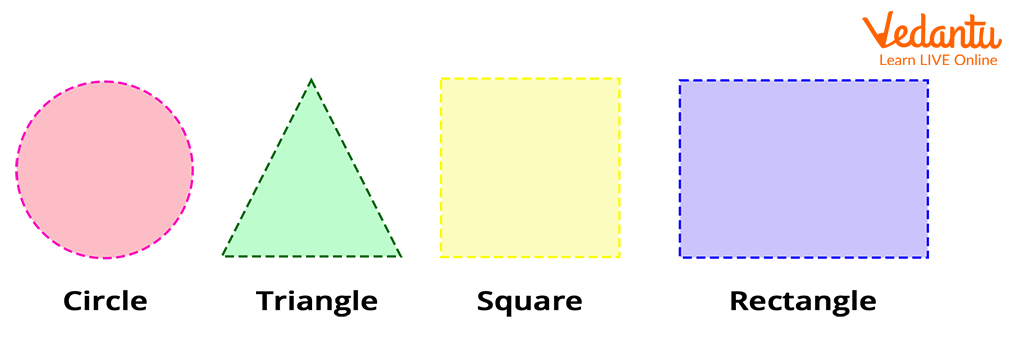2-D Shapes

### What are 3-D Shapes in Geometry?

Shapes that have three dimensions, i.e., length, width, and height are 3-D shapes. Below, you can find 3-D shapes: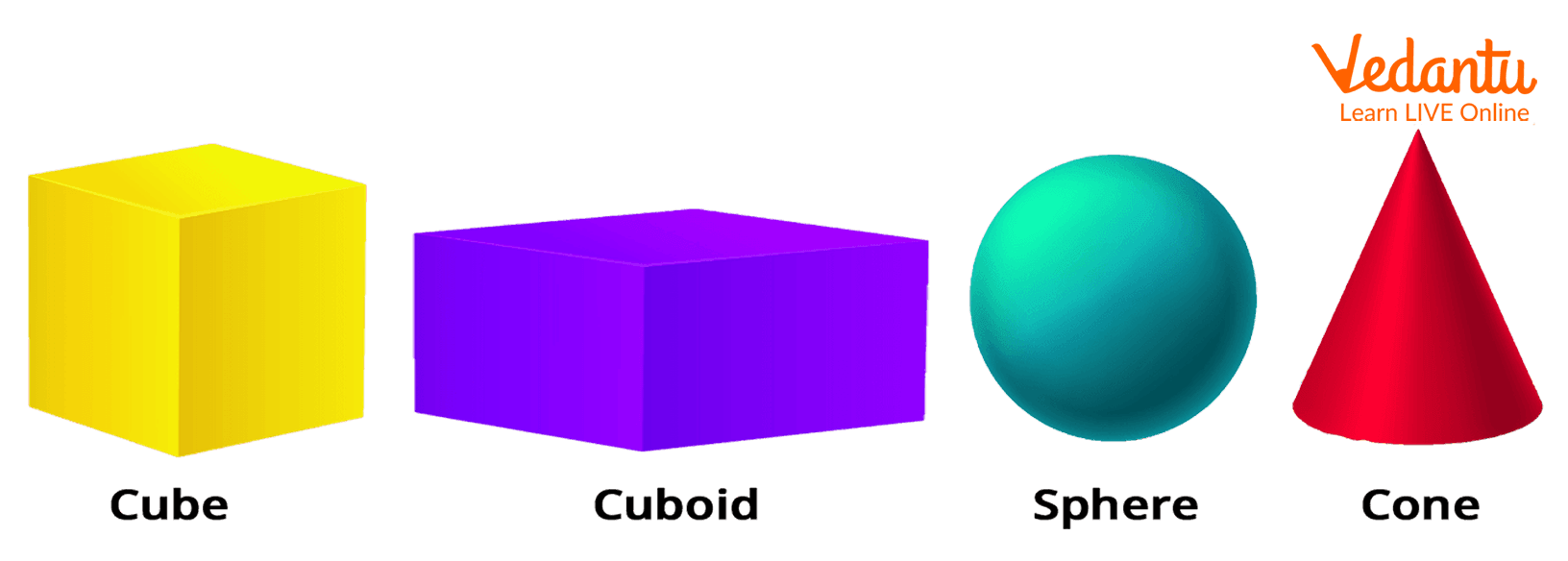3-D Shapes

All these shapes have respective parameters like a triangle has three edges, vertices, and angles. A cuboid has length, width, and height. Now, a question arises: what are these parameters? Well, all these terms come under Geometry Vocabulary and what does this vocabulary mean? Let us understand.

### Various Terms in Geometry

 Terms Description Point Point is a location represented by a dot or a position in space. Line A one-dimensional figure, which has length but no width. Segment (part) of a line. Ray In geometry, the ray is a part of a line that has a fixed starting point but no endpoint. However, it can extend infinitely in one direction. Besides this, on its way to infinity, a ray may pass through more than one point. Intersecting line segments For lines, rays, and line segments, the word intersect means to meet or cross. When two lines, rays, or line segments meet at a point, we call it an intersecting point. Parallel line segmentsWhen the two line segments do not cross each other, in other words they do not intersect (never meet) we call these segments as parallel line segments. Perpendicular line segmentsWhen two line segments stand in a way that they make a ‘T’ shape, such a type of arrangement is called perpendicular line segments. So, here one line segment is perpendicular or at right-angle or 90-degrees to another line segment. A square is formed by four line segments of equal length each, which means that the square has all four sides equal.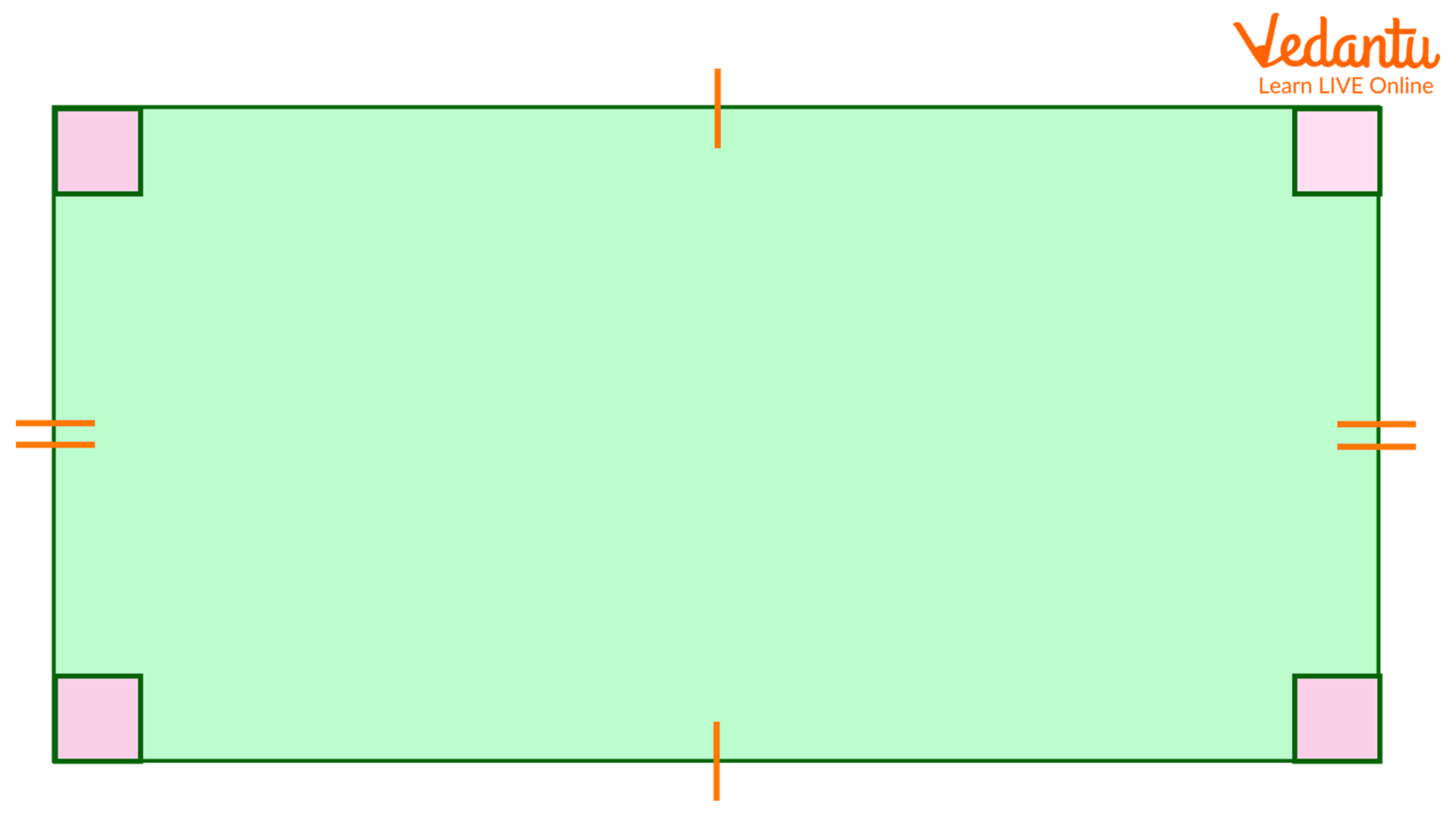A rectangle is a 2-D shape having four sides and four corners.Its two sides meet at right angles or 90-degrees, which means a rectangle has 4 angles, each measuring 90 ̊. Also, the opposite sides of a rectangle have the same lengths and are parallel.For example, unlike a square, two line segments are of say ‘20 m’ and the other two line segments are of ‘8 m’. So when joined, they form a rectangle. Triangle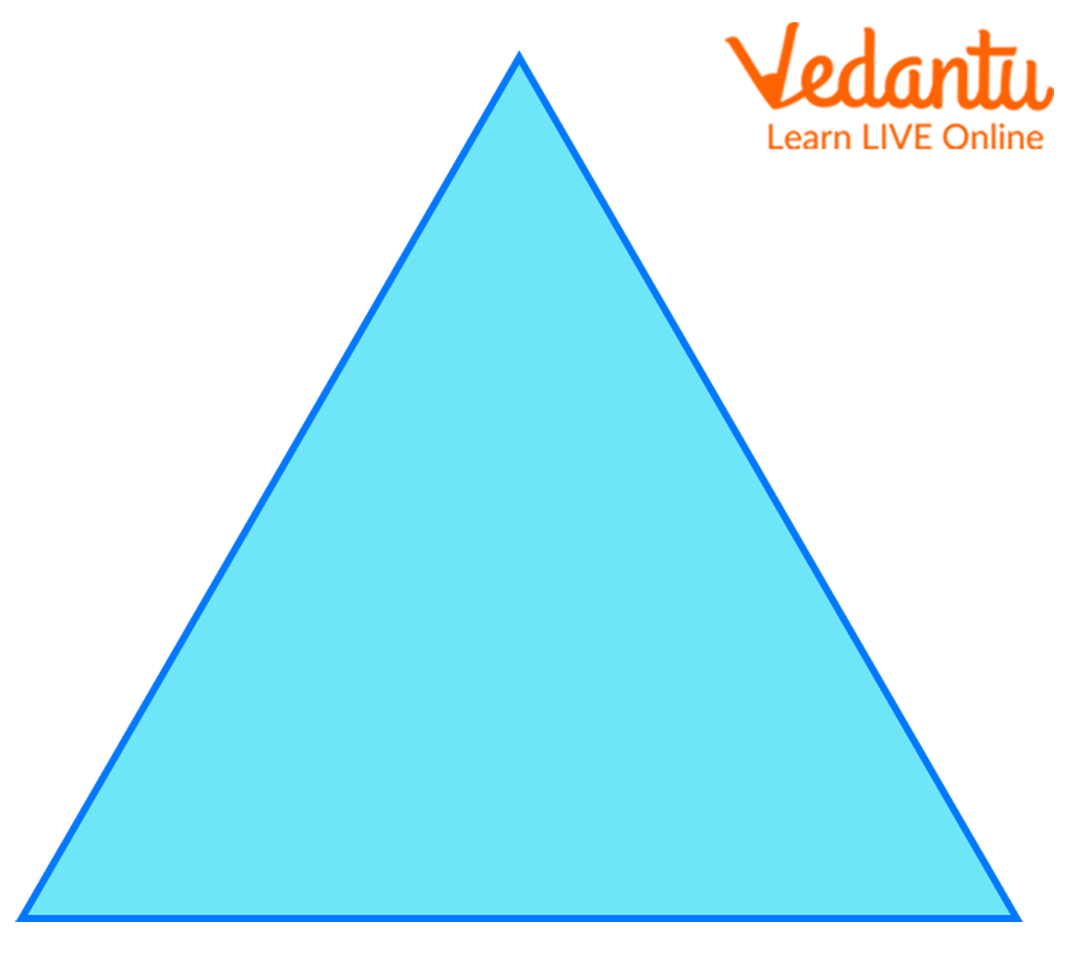A triangle is a polygon with three edges or sides (whose measure can/cannot be equal) and three vertices.These three line segments can be of equal length.Two segments can be of the same length, and the other different.It is also possible that all three line segments are of different lengths. Equilateral Triangle A triangle having all its sides equal is an equilateral triangle. Isosceles Triangle A triangle that has two sides of equal length while the third one is of a different length, we call this triangle an isosceles triangle.However, when a triangle has an angle of 90-degrees between two sides, it is a right-angled isosceles triangle. Scalene Triangle When all sides of a triangle are of different lengths, we call it a scalene triangle. Vertices (Singular: Vertex) A point where two or more line segments meet is called a vertex. And its plural form is vertices. Circle A 2-D shape that has no edge or side or a vertex.Though a circle doesn’t have any side, it has a circumference, diameter, and radius, which is denoted by ‘r’. Circumference The length of the line enclosing a circle is called its circumference. Radius Radius is defined as half of the diameter of a circle.The length of the line so formed is a diameter. When we half this length, it becomes the radius of a circle. Quadrilateral The word ‘Quad’ means four. Any polygon having four sides is a quadrilateral.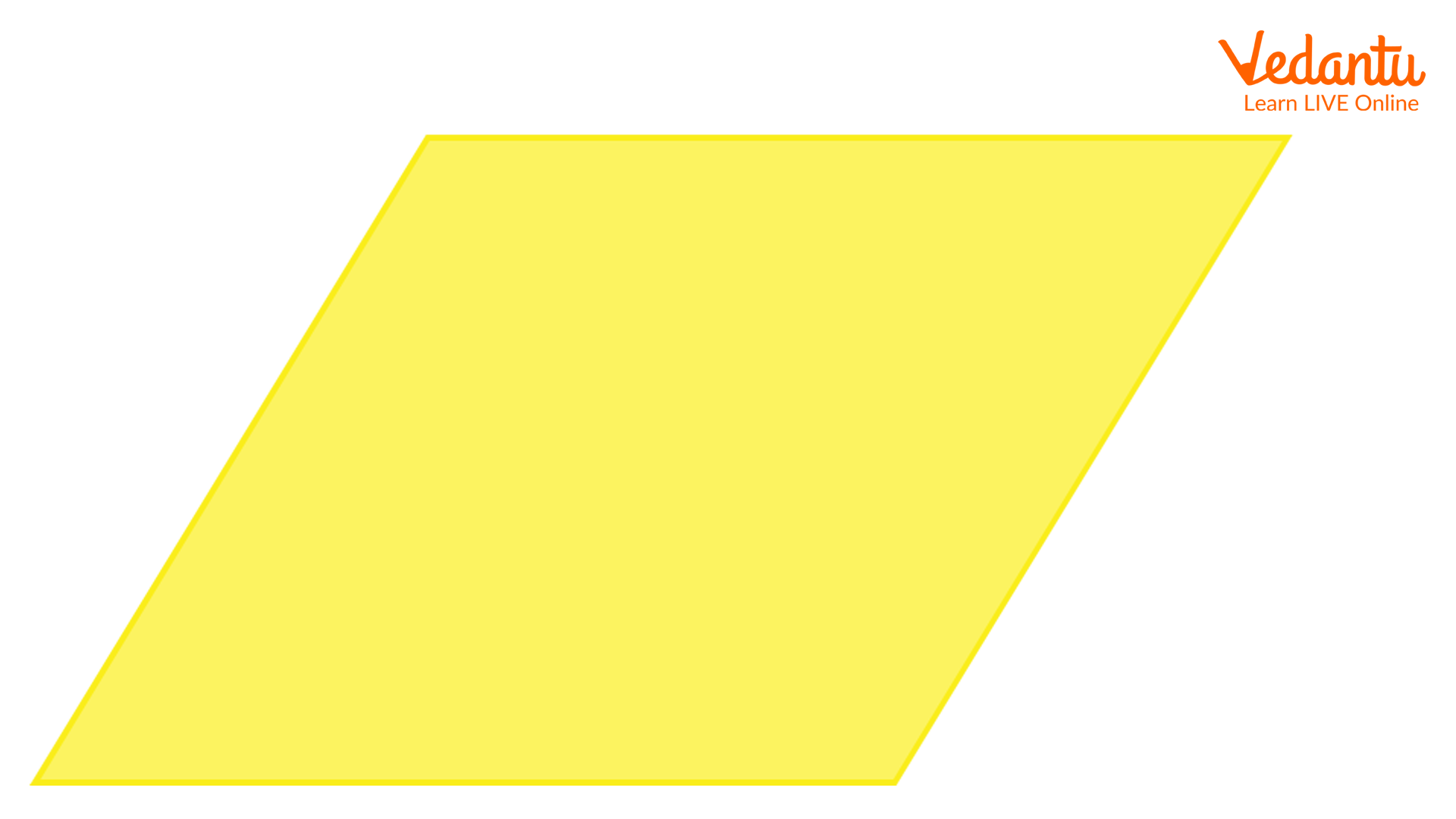Rhombus is a quadrilateral whose four sides have the same length. Angles An angle is the space between two lines or surfaces that meet, measured in degrees.These angles can be right, acute, and obtuse. Linear or 180-degree angle Look at your forehead and measure the angle, you notice that the angle is 180-degrees. Right Angle An angle that forms between two lines forming a T-shape is called a right-angle. Acute Angle Suppose that you have a T-shape made of two lines and let us tilt one line, you see that there is a change in the angle and that is less than 90-degrees.Thus, the angle formed here is an acute angle. Obtuse Angle When an angle is between 90 and 180-degrees, it is an obtuse angle.For example, if you tilt the ‘T-shape’ in such a way that the angle lies between 90-180, this shape has an obtuse angle.

So, this was all about the geometry vocabulary. Going through this will help you understand what parameters does a geometrical shape has.

Last updated date: 23rd Sep 2023
Total views: 138.6k
Views today: 1.38k

## FAQs on Geometry Vocabulary

1. List the polygons having more than 4 sides.

Following are the polygons having more than four sides.

• Pentagon

A shape that has five sides.

The number of vertices - 5

Internal angle measure - 108-degrees, therefore, the sum of internal angles - 5 x 108 = 540-degrees.

• Hexagon

A shape that has six sides and vertices.

Having six angles, where the sum of internal angles measure 720-degrees. However, the sum of external angles measures 360-degrees.

2. Define a trapezium and a parallelogram.

Trapezium- A quadrilateral that has two parallel and two nonparallel sides.

Parallelogram- It is a quadrilateral that has both pairs of opposite sides parallel to each other.